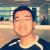# javascript中子类调用父类方法解决方案

OO设计的主旨和关于它的一些话题谈起来很大，但只着眼于Class的定义方式，我认为它是JavaScript开发者尝试解决问题的首选。因此，你可以在互联网上找到许多不同的问题解决案例，但在我看过它们后不免有些失望——这些案例都是在某个场合下适用，而不是放之四海而皆准的通法。而我对这个话题的兴趣来自于我的team在开发ThinWire Ajax Framework的影响。由于这个框架生成出对客户端代码的需求，才使我们“被迫”去实现可靠的、支持父类方法调用的OO模式。通过父类调用，你可以进一步依靠类的继承特性来核心化通用代码，从而更易于减少重复代码，去掉客户端代码的坏味道

More Solutions：

没有进行父类调用的简单继承:

`// 提前写好的JavaScript Class定义和继承// 当然，这种代码很丑陋，散发着代码的坏味道。function BaseClass() {    //BaseClass constructor code goes here }BaseClass.prototype.getName = function() {    return "BaseClass";}function SubClass() {    //SubClass constructor code goes here }//Inherit the methods of BaseClassSubClass.prototype = new BaseClass();//Override the parent's getName methodSubClass.prototype.getName = function() {    return "SubClass";}//Alerts "SubClass"alert(new SubClass().getName());`

`// 运行时的JavaScript Class 定义和继承// 看上去很传统，但这些脚本会导致在Internet Explorer中的内存泄漏.function BaseClass() {    this.getName = function() {        return "BaseClass";    };        //BaseClass constructor code goes here }function SubClass() {    //在对象实例建立时重载父类的getName方法     this.getName = function() {        return "SubClass";    }    //SubClass constructor code goes here }//Inherit the methods of BaseClassSubClass.prototype = new BaseClass();//Alerts "SubClass"alert(new SubClass().getName());`

`function BaseClass() { }BaseClass.prototype.getName = function() {    return "BaseClass(" + this.getId() + ")";}BaseClass.prototype.getId = function() {    return 1;}function SubClass() {}SubClass.prototype = new BaseClass();SubClass.prototype.getName = function() {    //调用父类的getName()方法    //哈哈，这是对父类调用的直接引用吗？    return "SubClass(" + this.getId() + ") extends " +        BaseClass.prototype.getName();}SubClass.prototype.getId = function() {    return 2;}//输出结果："SubClass(2) extends BaseClass(1)";//这是正确的输出吗?alert(new SubClass().getName());`

`function BaseClass() { }BaseClass.prototype.getName = function() {    return "BaseClass(" + this.getId() + ")";}BaseClass.prototype.getId = function() {    return 1;}function SubClass() {}SubClass.prototype = new BaseClass();SubClass.prototype.getName = function() {    //一点魔法加上多态性！    //但很明显，这还是一个直接引用!        return "SubClass(" + this.getId() + ") extends " +        BaseClass.prototype.getName.call(this);}SubClass.prototype.getId = function() {    return 2;}//输出结果："SubClass(2) extends BaseClass(2)";//Hey, 我们得到了正确的输出!alert(new SubClass().getName());`

ECMA-262 JavaScript/EcmaScript标准中， Call()方法是所有Function实例的一个成员方法，这已经被所有的主流浏览器所支持。JavaScript把所有的function看作对象，因此每个function都具有方法和附着其上的属性。Call()方法允许你调用某个function，并在function的调用过程中确定 “this”变量应该是什么。JavaScript的function没有被紧紧地绑定到它所在的对象上，所以如果你没有显式地使用call()方法， “this”变量将成为function所在的对象。

Douglas Crockford的Solution:

JavaScript没有提供对通过“隐性引用”方式调用父类方法的支持，这里也没有在其它OO语言中使用的“super”变量的等价物。于是，一些开发者做出了自己的解决方案，但就像我前面提到的那样，每个解决方案都存在某种缺点。

Douglas Crockford的方法在多数情况下可以正常工作:

`//Crockford的方法：给所有的function都增加'inherits' 方法、//每个类都增加了'uber'方法来调用父类方法Function.prototype.inherits = function(parent) {    var d = 0, p = (this.prototype = new parent());        this.prototype.uber = function(name) {        var f, r, t = d, v = parent.prototype;        if (t) {            while (t) {                v = v.constructor.prototype;                t -= 1;            }            f = v[name];        } else {            f = p[name];            if (f == this[name]) {                f = v[name];            }        }        d += 1;        r = f.apply(this, Array.prototype.slice.apply(arguments, ));        d -= 1;        return r;    };};`

`function BaseClass() { }BaseClass.prototype.getName = function() {    return "BaseClass(" + this.getId() + ")";}BaseClass.prototype.getId = function() {    return 1;}function SubClass() {}SubClass.inherits(BaseClass);SubClass.prototype.getName = function() {    //这里看上去非常的清晰，它调用了BaseClass的getName()方法return "SubClass(" + this.getId() + ") extends " +        this.uber("getName");}SubClass.prototype.getId = function() {    return 2;}function TopClass() {}TopClass.inherits(SubClass);TopClass.prototype.getName = function() {    //这里看上去非常的清晰，它调用了SubClass的getName()方法    return "TopClass(" + this.getId() + ") extends " +        this.uber("getName");}TopClass.prototype.getId = function() {    //Ok, 因此this.getId()应该总是//返回调用SubClass的getId()方法的返回值（2）。    return this.uber("getId");}//输出结果："TopClass(2) extends SubClass(1) extends BaseClass(1)"//嗯？后面的两次this.getId()调用都没有返回2.//发生了什么？ alert(new TopClass().getName());`

`//定义最顶级类function Class() { }Class.prototype.construct = function() {};Class.__asMethod__ = function(func, superClass) {        return function() {        var currentSuperClass = this.\$;        this.\$ = superClass;        var ret = func.apply(this, arguments);                this.\$ = currentSuperClass;        return ret;    };};Class.extend = function(def) {    var classDef = function() {        if (arguments !== Class) { this.construct.apply(this, arguments); }    };        var proto = new this(Class);    var superClass = this.prototype;        for (var n in def) {        var item = def[n];                                        if (item instanceof Function) {            item = Class.__asMethod__(item, superClass);        }                proto[n] = item;    }    proto.\$ = superClass;    classDef.prototype = proto;        //赋给这个新的子类同样的静态extend方法    classDef.extend = this.extend;            return classDef;};`

`//Hey, 注意一下这个类的定义方式//看上去比其它方式要清楚些var BaseClass = Class.extend({    construct: function() { /* optional constructor method */ },        getName: function() {        return "BaseClass(" + this.getId() + ")";    },        getId: function() {        return 1;    }});var SubClass = BaseClass.extend({    getName: function() {        //调用BaseClass的getName()方法        return "SubClass(" + this.getId() + ") extends " +            this.\$.getName.call(this);    },        getId: function() {        return 2;    }});var TopClass = SubClass.extend({    getName: function() {        //调用SubClass的getName()方法        return "TopClass(" + this.getId() + ") extends " +            this.\$.getName.call(this);    },        getId: function() {        //this.getId()总是返回调用父类的getId()方法的返回值（2）        return this.\$.getId.call(this);    }});//输出结果："TopClass(2) extends SubClass(2) extends BaseClass(2)"//一切都正确!alert(new TopClass().getName());`

`//定义最顶级类function Class() { }Class.prototype.construct = function() {};Class.extend = function(def) {    var classDef = function() {        if (arguments !== Class) { this.construct.apply(this, arguments); }    };        var proto = new this(Class);    var superClass = this.prototype;        for (var n in def) {        var item = def[n];                                if (item instanceof Function) item.\$ = superClass;        proto[n] = item;    }    classDef.prototype = proto;        //赋给这个新的子类同样的静态extend方法     classDef.extend = this.extend;            return classDef;};`

`//Hey, 注意一下这个类的定义方式//看上去比其它方式要清楚些var BaseClass = Class.extend({    construct: function() { /* optional constructor method */ },            getName: function() {        return "BaseClass(" + this.getId() + ")";    },        getId: function() {        return 1;    }});var SubClass = BaseClass.extend({    getName: function() {        //调用BaseClass的getName()方法        return "SubClass(" + this.getId() + ") extends " +            arguments.callee.\$.getName.call(this);    },        getId: function() {        return 2;    }});var TopClass = SubClass.extend({    getName: function() {        //调用SubClass的getName()方法        return "TopClass(" + this.getId() + ") extends " +            arguments.callee.\$.getName.call(this);    },        getId: function() {        // this.getId()总是返回调用父类的getId()方法的返回值（2）         return arguments.callee.\$.getId.call(this);    }});//输出结果："TopClass(2) extends SubClass(2) extends BaseClass(2)"//工作正常！而且没有任何中间functionalert(new TopClass().getName());`

00#### 引用来自#2楼“艺术家”的帖子

0